# Test Prep Plan - Take a practice test

Take this practice test to check your existing knowledge of the course material. We'll review your answers and create a Test Prep Plan for you based on your results.
How Test Prep Plans work
1
2Based on your results, we'll create a customized Test Prep Plan just for you!
3Study smarter
Study more effectively: skip concepts you already know and focus on what you still need to learn.

# NMTA Middle Grades Math: Properties of Functions Chapter Exam

Exam Instructions:

Choose your answers to the questions and click 'Next' to see the next set of questions. You can skip questions if you would like and come back to them later with the yellow "Go To First Skipped Question" button. When you have completed the practice exam, a green submit button will appear. Click it to see your results. Good luck!

### Page 2

#### Question 9 9. In the figure shown below, the graph of the yellow function is a translation of the graph of the blue function. If the expression for the blue function is y(x) = b(x), which of the following is the equation of the yellow function?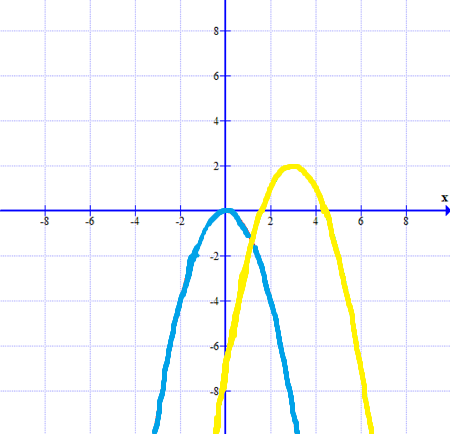### Page 3

#### Question 13 13. What is the domain of the function below?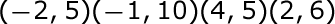#### Question 15 15. If the graph of f(x) is shown below, which choice represents the graph of f(x + 1) + 2?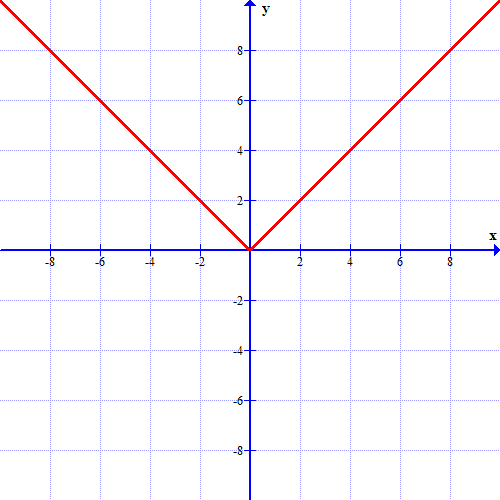### Page 6

#### Question 26 26. What is the range of the function represented by the graph below?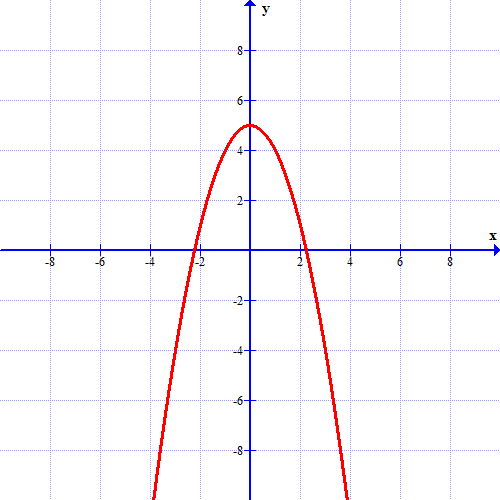#### Question 27 27. Which kind of transformation would change the purple function below into the yellow one?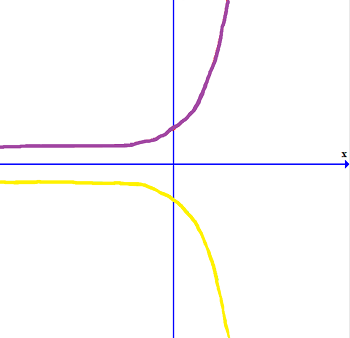#### Question 28 28. What is the inverse function of f(x) = 3(x - 2)3?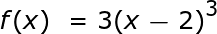#### Question 29 29. Find the domain of f(x)/g(x), if: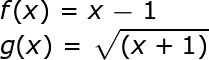#### Question 30 30. Using the graph, find f(f(-2)).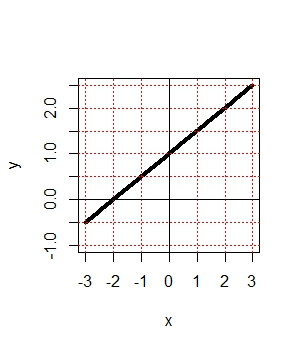#### NMTA Middle Grades Math: Properties of Functions Chapter Exam Instructions

Choose your answers to the questions and click 'Next' to see the next set of questions. You can skip questions if you would like and come back to them later with the yellow "Go To First Skipped Question" button. When you have completed the practice exam, a green submit button will appear. Click it to see your results. Good luck!

Support